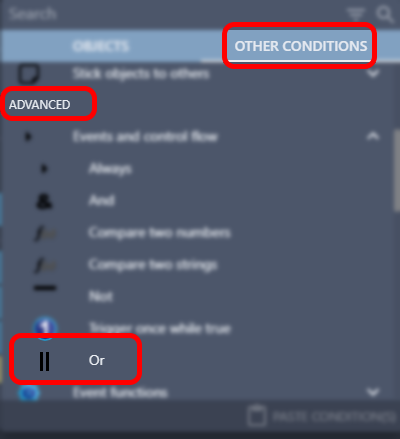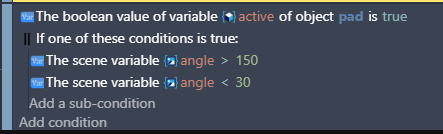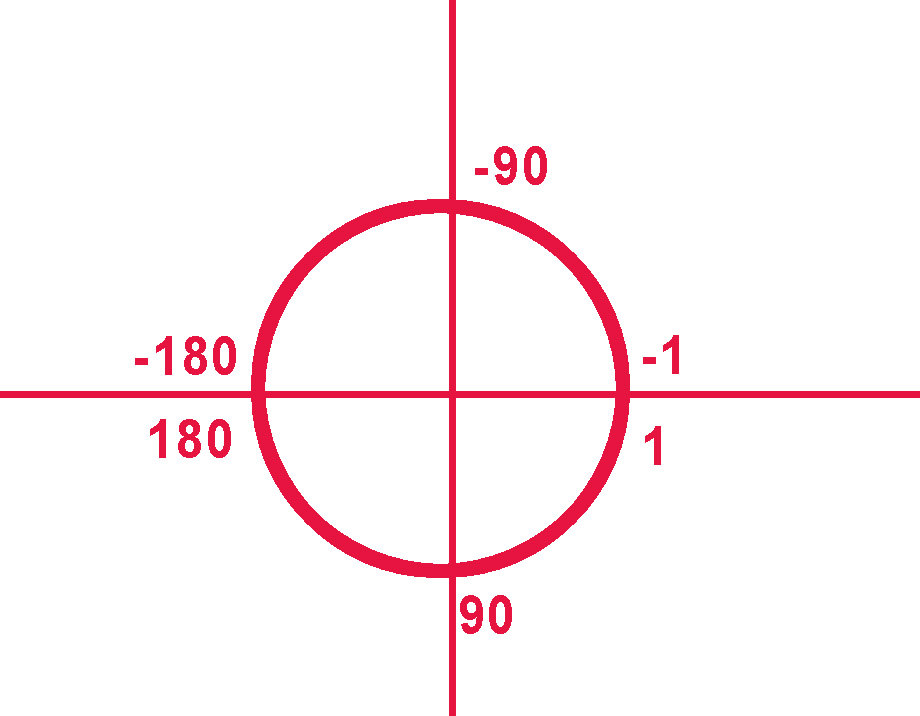# [Solved] Two angle conditions don't work

two angle conditions don’t work
but when I break them in seperate
then it works
I don’t know
is it a bug ?

Well, yes, it won’t work because having those conditions like that is saying all 3 conditions must be true. And you can’t have a number greater than 150 and less than 30.

You want to use an OR condition, and have the 2 angle checks within it :to get :ok, thanks, SOLVED, that will work for mebut that two conditions should not have be anti complementary

I mean angle from 30 to 150 should work ? but it doesn’tMost angles are tracked in degrees, which goes from 0 (starting at the right) and going up to 360.

Very few things in the system are tracked from 0 throifh 180/-1 through -180.

But even if it was, you were saying it had to be BOTH above 150 and below 30 at the same time. Which isn’t mathematically possible. The math is normal math. Even if the angle loops, the math doesn’t.

I’m not 100% sure on what your question is.

As mentioned, while the angle may go negative, the math in your events will not.

So 30 < X < 150 would mean
“If angle is > 30” and “If angle is < 150” So only 30.01 through 149.999999 would be valid.
Your screenshot in the original post wasn’t asking for this. It was asking for “If angle is < 30” (lower than 30) and “If angle is > 150” (greater than 150), or (x < 30 and 150 < x). No number can meet this requirement.

MrMen’s post above would be correct for 30.01 through 149.99999. There is no number that will be greater than 150 and less than 30 at the same time.